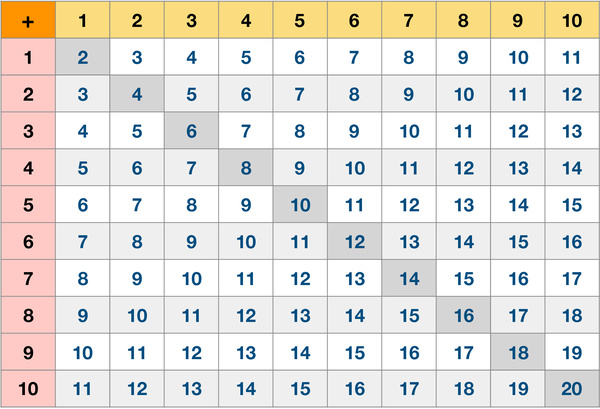# Addition Table 10 by 10Addition, subtraction, multiplication and division are the four elementary mathematical operations of arithmetic. Around 6 years old, most kids are ready to learn facts about addition. Addition is the first operation kids learn. Mastery of the small number addition facts is the foundation for learning other elementary arithmetic operations. There are many ways parents can work with kids to help them learn addition.

This worksheet provides a table of equations showing the sums from 1 + 1 up to 10 + 10. Memorizing the addition table is an important skill for learning mathematics. Many educators believe it is necessary to memorize this table. With the help of this table, parents could help kids learn, memorize, practice and recall the facts of adding small numbers easily. It is also useful to explore patterns on the table to help kids memorize the facts.